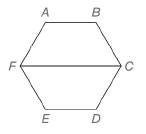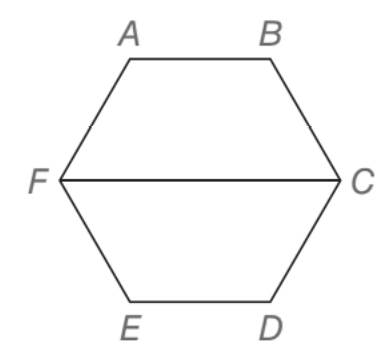Chapter 4.2, Problem 38EElementary Geometry For College St...

7th Edition
Alexander + 2 others
ISBN: 9781337614085

Solutions

Chapter
SectionElementary Geometry For College St...

7th Edition
Alexander + 2 others
ISBN: 9781337614085
Textbook Problem

For regular hexagon A B C D E F ,a) are quadrilateral A B C F and quadrilateral E D C F congruent?b) is F C ¯ parallel to A B ¯ and also to E D ¯ ?To determine

a)

Whether the quadrilateral ABCF and quadrilateral EDCF are congruent.

Explanation

Given,

ABCDEF is a hexagon.

Here, AB¯BC¯CD¯DE¯EF¯FA¯

To determine

b) Whether FC¯ parallel to AB¯ and also to ED¯.ABCDEF is a hexagon.

Still sussing out bartleby?

Check out a sample textbook solution.

See a sample solution

The Solution to Your Study Problems

Bartleby provides explanations to thousands of textbook problems written by our experts, many with advanced degrees!

Get Started

Evaluate the integrals in Problems 5-28. Check your answers by differentiating. 19.

Mathematical Applications for the Management, Life, and Social Sciences

Proof Prove Theorem 11.9.

Calculus: Early Transcendental Functions

Use an integral to estimate the sum i=110000i.

Single Variable Calculus: Early Transcendentals

limx0+(1+x)1/x= a) e b) e c) 1e d) 1e

Study Guide for Stewart's Single Variable Calculus: Early Transcendentals, 8th

(i · j)k = k 2-k 0 (the number 0) 0 (the zero vector)

Study Guide for Stewart's Multivariable Calculus, 8th